Like   Tweet
 /* styles */ May 20, 2020 Dear Readers, This is the seventh installment of “Expand Your Horizons While Sheltering at Home” from the Dayton Literary Peace Prize. The DLPP class of 2013 provides an especially rich reading experience. War dominates fiction and nonfiction provides some antidotes to war. The Korean War takes center stage in three of the books, the Arabic wars appear in two, World War II in two other works, while the rest lead us to understand how cultural divisions can push a brutalized people to the brink of war. We see that shove toward violence in the Ojibwa clan as tribal law and white man’s law clash in a story told from the eyes of a young boy; in Romania, we see a young girl traumatized by her father’s arrest for political dissidence; we see a young lacemaker from Belfast come to terms with the horrors of Berlin; we watch in fear as four young black men’s lives are destroyed by white man’s lawlessness in Florida, and we hold our breath as a young man escapes from North Korea. But there is redemption even in war and we also see how diverse communities can live in peace. When we see children of all exceptionalities who have fallen far from the tree be accepted, we understand all people can come to be accepted. In the end, Wendell Berry brings us home through the prose we celebrated in 2013, and through his poetry. We take special solace from his poem The Peace of Wild Things. When despair for the world grows in me and I wake in the night at the least sound in fear of what my life and my children’s lives may be, I go and lie down where the wood drake rests in his beauty on the water, and the great heron feeds. I come into the peace of wild things who do not tax their lives with forethought of grief. I come into the presence of still water. And I feel above me the day-blind stars waiting with their light. For a time I rest in the grace of the world, and am free. Stay safe. Be well. Read books.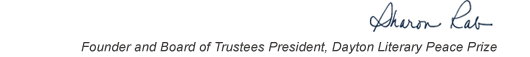table div table+table+table+table div table{width:100%;padding:0}table div table+table+table+table div table img{width:96.23%;padding:0;float:none}table div table+table+table+table div table td{width:100%;padding:0 1.88% 18px}/* styles */This list includes the winners and runners-up for 2013 and we have rich background information on each one on our website.

▪ Under Past Winners. scroll down to the year and click on it.
▪ You will have the option of clicking on a winner, a runner-up, or the finalists.
 ▪ Under Past Winners. scroll down to the year and click on it.
 ▪ You will have the option of clicking on a winner, a runner-up, or the finalists.

On the bottom right of the home page, you can click on COREScholar, which is built by the Chair of our Curriculum Committee Carol Loranger, Associate Dean of the College of Liberal Arts at Wright State, and maintained for us by Wright State University. There you can find interviews, TED Talks, articles, other books, films, etc. by and about each of our winning and runner-up authors. It is a great resource for students, book clubs, and readers who would like to explore an author in depth.

 table div table+table+table+table+table+table div table{width:100%;padding:0}table div table+table+table+table+table+table div table img{width:96.23%;padding:0;float:none}table div table+table+table+table+table+table div table td{width:100%;padding:0 1.88% 18px}/* styles *//* styles */ “Read global; buy local.” — Marlon James, DLPP 2009 Fiction Winner for The Book of Night Women
 table div table+table+table+table+table+table+table+table div table{width:100%;padding:0}table div table+table+table+table+table+table+table+table div table img{width:96.23%;padding:0;float:none}table div table+table+table+table+table+table+table+table div table td{width:100%;padding:0 1.88% 18px}/* styles */## 2013 Finalists - Fiction

 table div table+table+table+table+table+table+table+table+table+table div table{width:100%;padding:0}table div table+table+table+table+table+table+table+table+table+table div table img{width:96.23%;padding:0;float:none}table div table+table+table+table+table+table+table+table+table+table div table td{width:100%;padding:0 1.88% 18px}/* styles */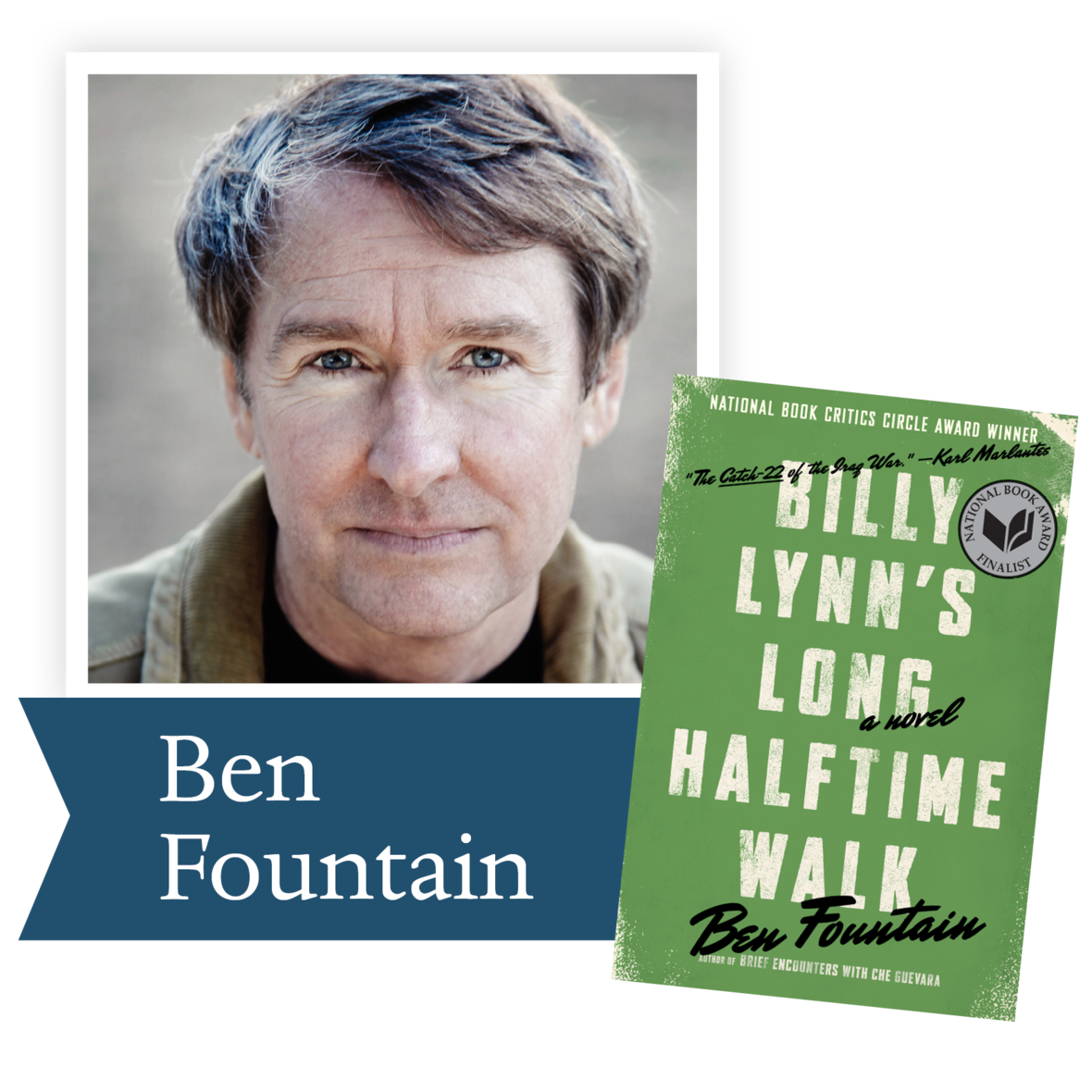Billy Lynn’s Long Halftime Walk
by Ben Fountain (HarperCollins): A hilarious
and heartbreaking day unfolds in the life of an Iraq War hero whose squad appears in a Dallas Cowboys halftime show as part of an effort to rekindle support for the war.

 table div table+table+table+table+table+table+table+table+table+table+table+table div table{width:100%;padding:0}table div table+table+table+table+table+table+table+table+table+table+table+table div table img{width:96.23%;padding:0;float:none}table div table+table+table+table+table+table+table+table+table+table+table+table div table td{width:100%;padding:0 1.88% 18px}/* styles */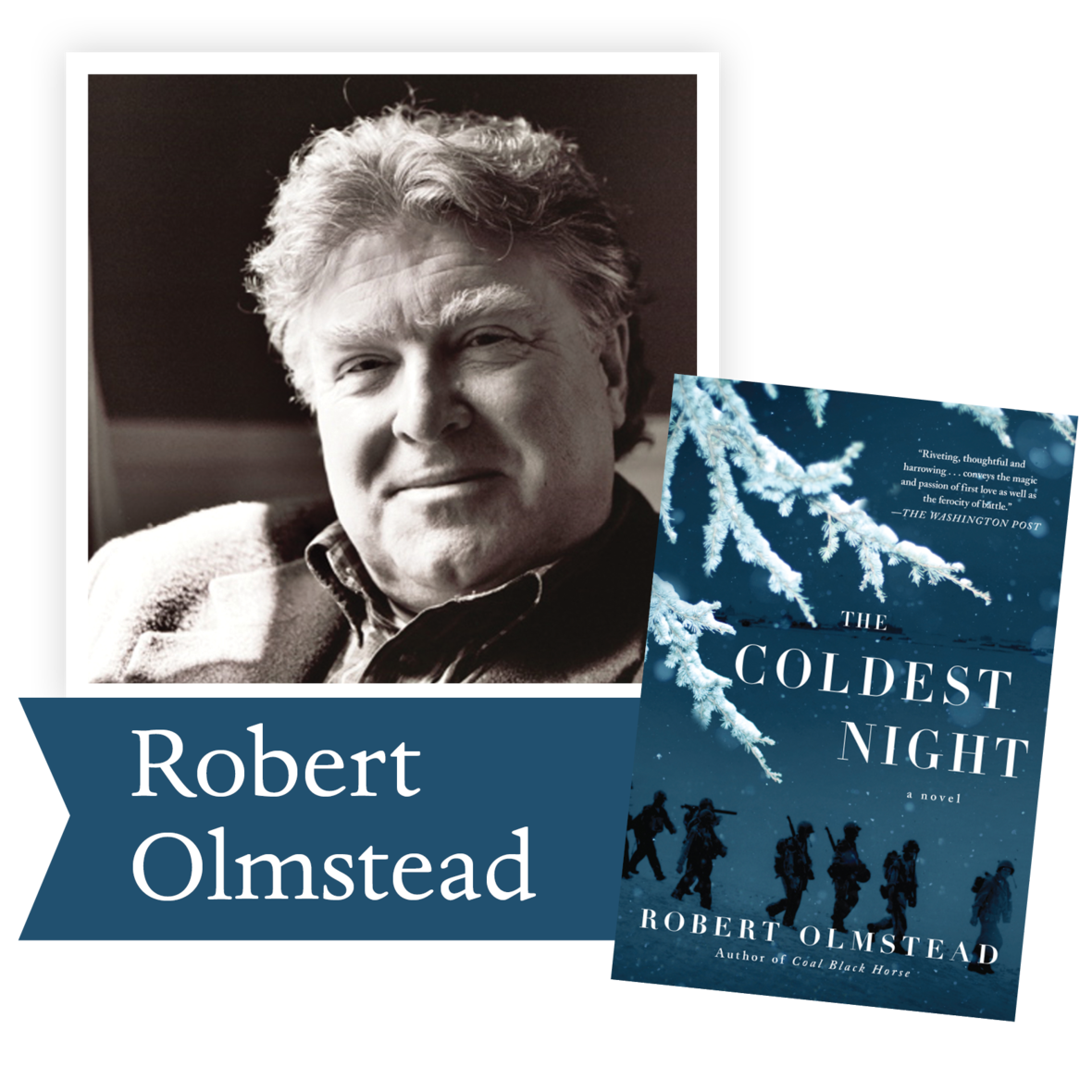The Coldest Night by Robert Olmstead
(Algonquin): A mesmerizing coming-of-age
novel that moves from the steamy streets of New Orleans to one of the most physically challenging battles in the Korean War.

 table div table+table+table+table+table+table+table+table+table+table+table+table+table+table div table{width:100%;padding:0}table div table+table+table+table+table+table+table+table+table+table+table+table+table+table div table img{width:96.23%;padding:0;float:none}table div table+table+table+table+table+table+table+table+table+table+table+table+table+table div table td{width:100%;padding:0 1.88% 18px}/* styles */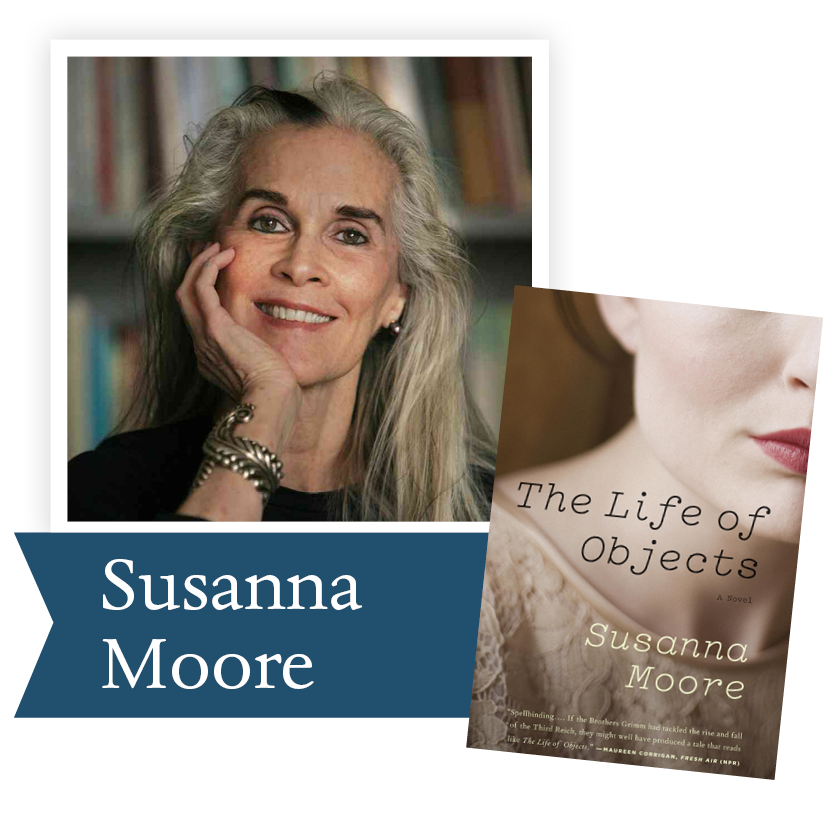The Life of Objects by Susanna Moore
(Random House): In 1938 Belfast, a young lace maker is whisked away from her dreary life to a glamorous Berlin household, only to find her fairy tale shattered by the realities of encroaching war.

 table div table+table+table+table+table+table+table+table+table+table+table+table+table+table+table+table div table{width:100%;padding:0}table div table+table+table+table+table+table+table+table+table+table+table+table+table+table+table+table div table img{width:96.23%;padding:0;float:none}table div table+table+table+table+table+table+table+table+table+table+table+table+table+table+table+table div table td{width:100%;padding:0 1.88% 18px}/* styles */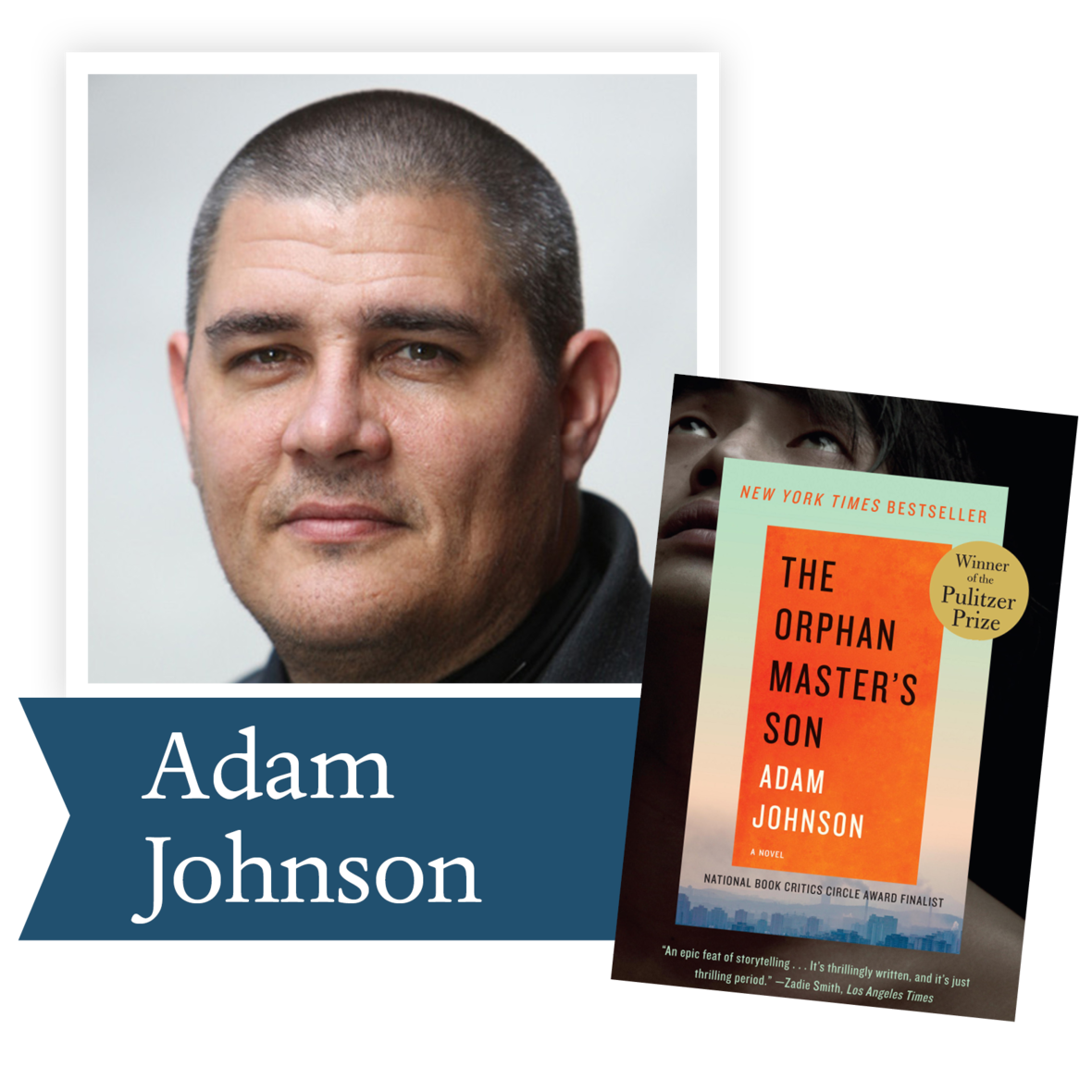The Orphan Master’s Son by Adam Johnson (Random House): In this Pulitzer Prize-winning novel, Adam Johnson provides a riveting portrait of a world rife with hunger, corruption, and casual cruelty but also camaraderie and stolen moments of beauty and love.

 table div table+table+table+table+table+table+table+table+table+table+table+table+table+table+table+table+table+table div table{width:100%;padding:0}table div table+table+table+table+table+table+table+table+table+table+table+table+table+table+table+table+table+table div table img{width:96.23%;padding:0;float:none}table div table+table+table+table+table+table+table+table+table+table+table+table+table+table+table+table+table+table div table td{width:100%;padding:0 1.88% 18px}/* styles */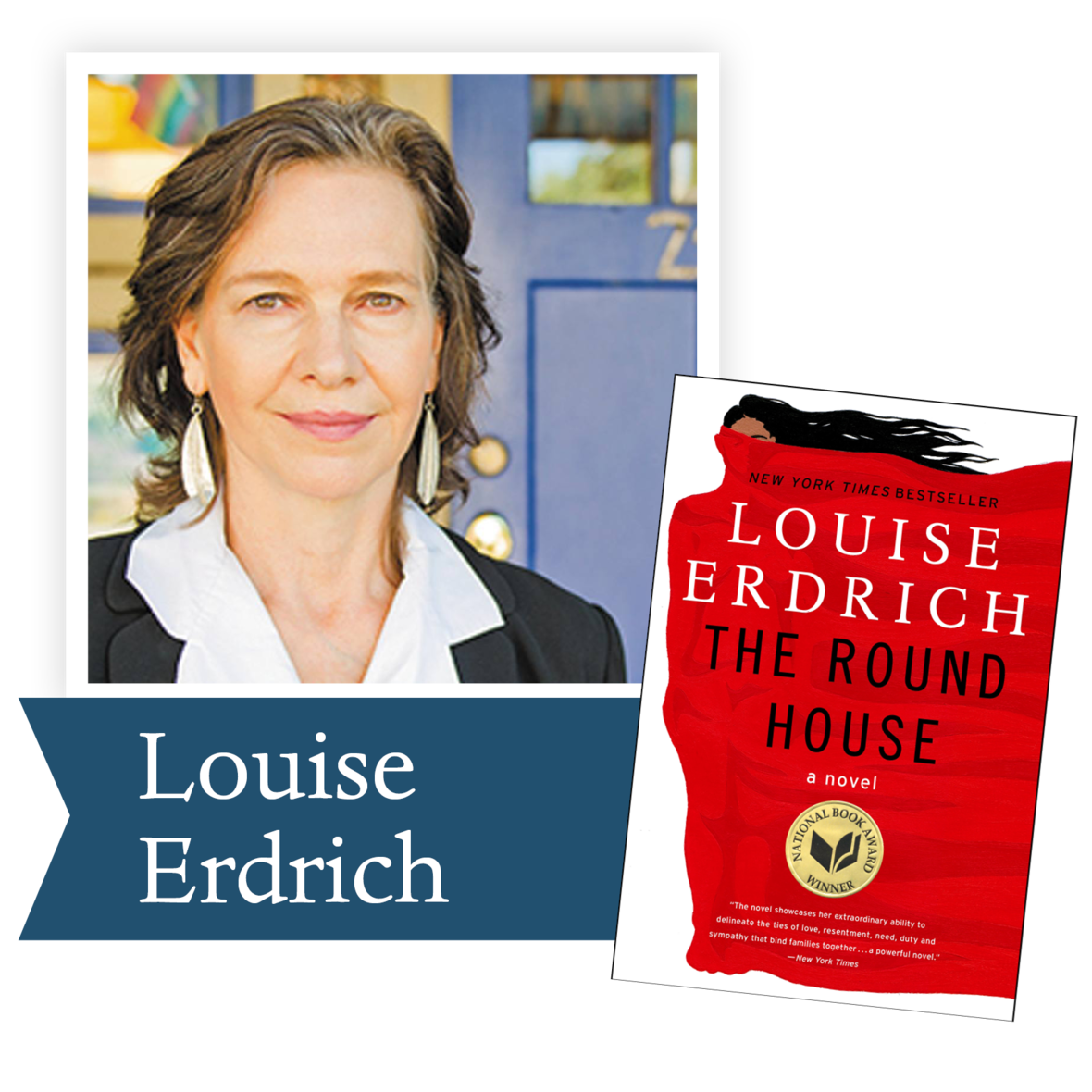The Round House by Louise Erdrich
(HarperCollins): A 13-year-old boy living on
an Ojibwe reservation in North Dakota sets out with three friends on a quest for answers about an attack on his mother that has left her too traumatized to leave her bed.

 table div table+table+table+table+table+table+table+table+table+table+table+table+table+table+table+table+table+table+table+table div table{width:100%;padding:0}table div table+table+table+table+table+table+table+table+table+table+table+table+table+table+table+table+table+table+table+table div table img{width:96.23%;padding:0;float:none}table div table+table+table+table+table+table+table+table+table+table+table+table+table+table+table+table+table+table+table+table div table td{width:100%;padding:0 1.88% 18px}/* styles */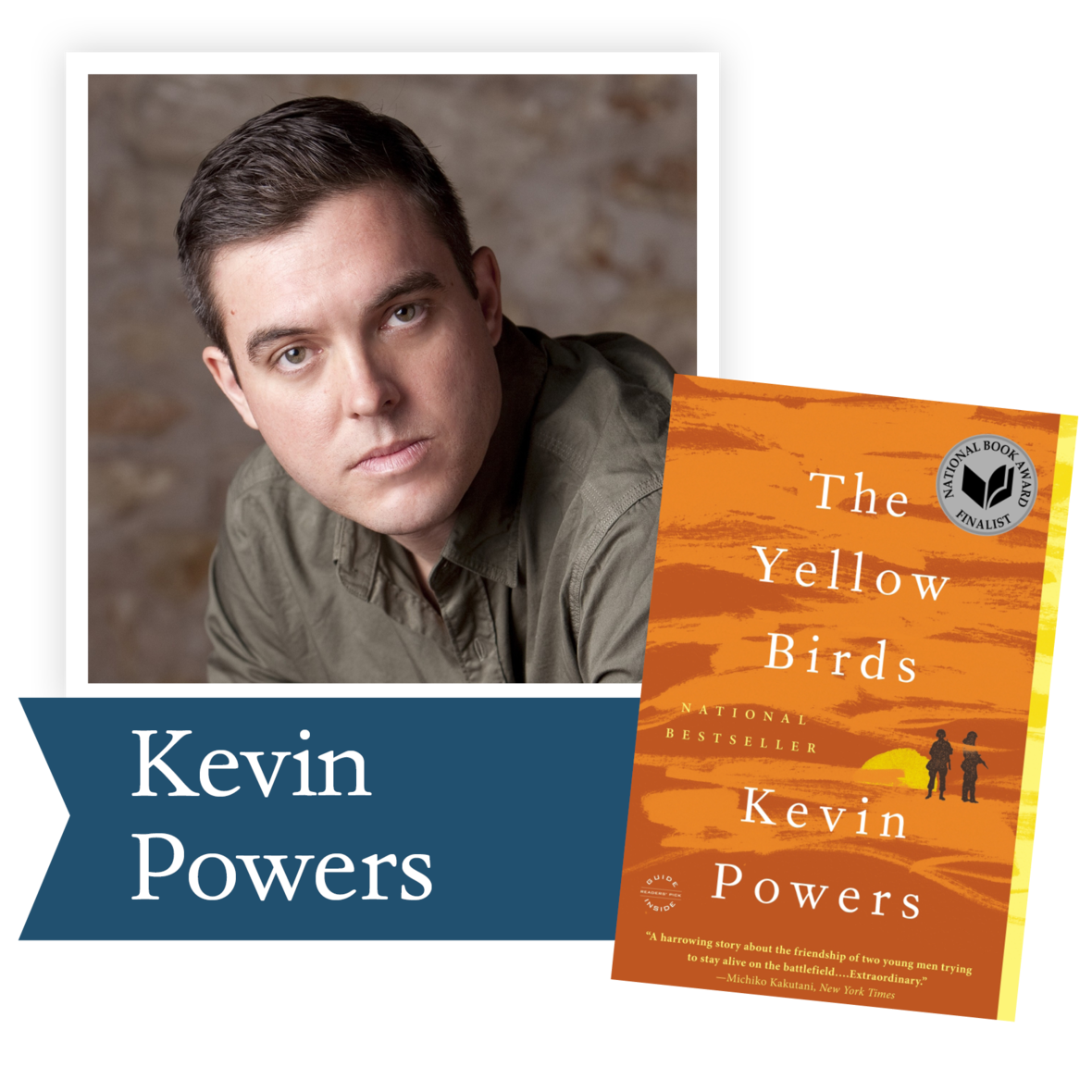The Yellow Birds by Kevin Powers
(Little, Brown and Company): Praised by Tom Wolfe as “the All Quiet on the Western Front of America’s Arab wars,” this bestselling debut novel by an Iraq War veteran recounts a bloody battle through the eyes of two young soldiers.

 table div table+table+table+table+table+table+table+table+table+table+table+table+table+table+table+table+table+table+table+table+table+table div table{width:100%;padding:0}table div table+table+table+table+table+table+table+table+table+table+table+table+table+table+table+table+table+table+table+table+table+table div table img{width:96.23%;padding:0;float:none}table div table+table+table+table+table+table+table+table+table+table+table+table+table+table+table+table+table+table+table+table+table+table div table td{width:100%;padding:0 1.88% 18px}/* styles */## 2013 Finalists - Nonfiction

 table div table+table+table+table+table+table+table+table+table+table+table+table+table+table+table+table+table+table+table+table+table+table+table+table div table{width:100%;padding:0}table div table+table+table+table+table+table+table+table+table+table+table+table+table+table+table+table+table+table+table+table+table+table+table+table div table img{width:96.23%;padding:0;float:none}table div table+table+table+table+table+table+table+table+table+table+table+table+table+table+table+table+table+table+table+table+table+table+table+table div table td{width:100%;padding:0 1.88% 18px}/* styles */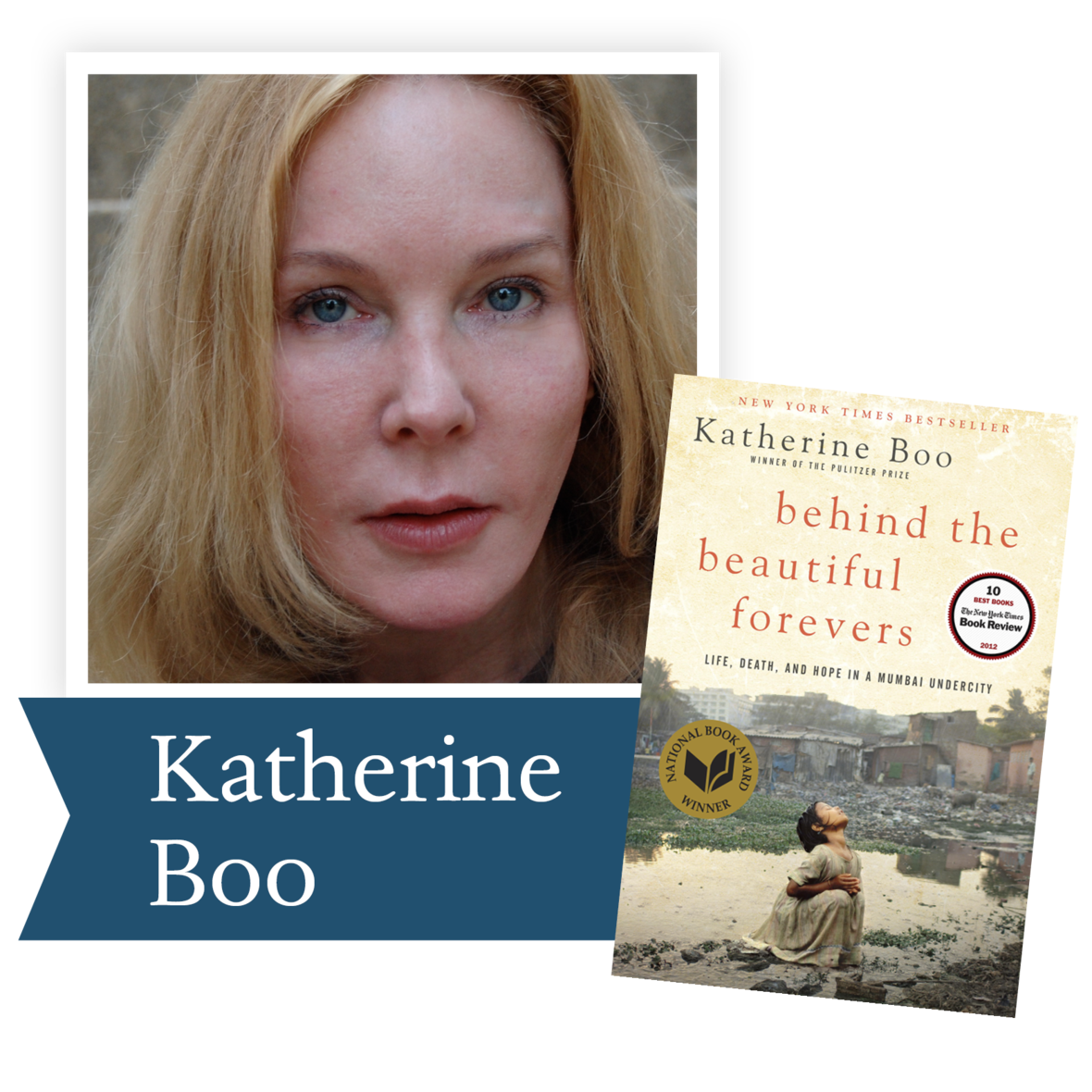Behind the Beautiful Forevers by Katherine Boo (Random House): Global change and inequality are given human faces via the residents of a makeshift settlement in the shadow of Mumbai’s luxury hotels.

 table div table+table+table+table+table+table+table+table+table+table+table+table+table+table+table+table+table+table+table+table+table+table+table+table+table+table div table{width:100%;padding:0}table div table+table+table+table+table+table+table+table+table+table+table+table+table+table+table+table+table+table+table+table+table+table+table+table+table+table div table img{width:96.23%;padding:0;float:none}table div table+table+table+table+table+table+table+table+table+table+table+table+table+table+table+table+table+table+table+table+table+table+table+table+table+table div table td{width:100%;padding:0 1.88% 18px}/* styles */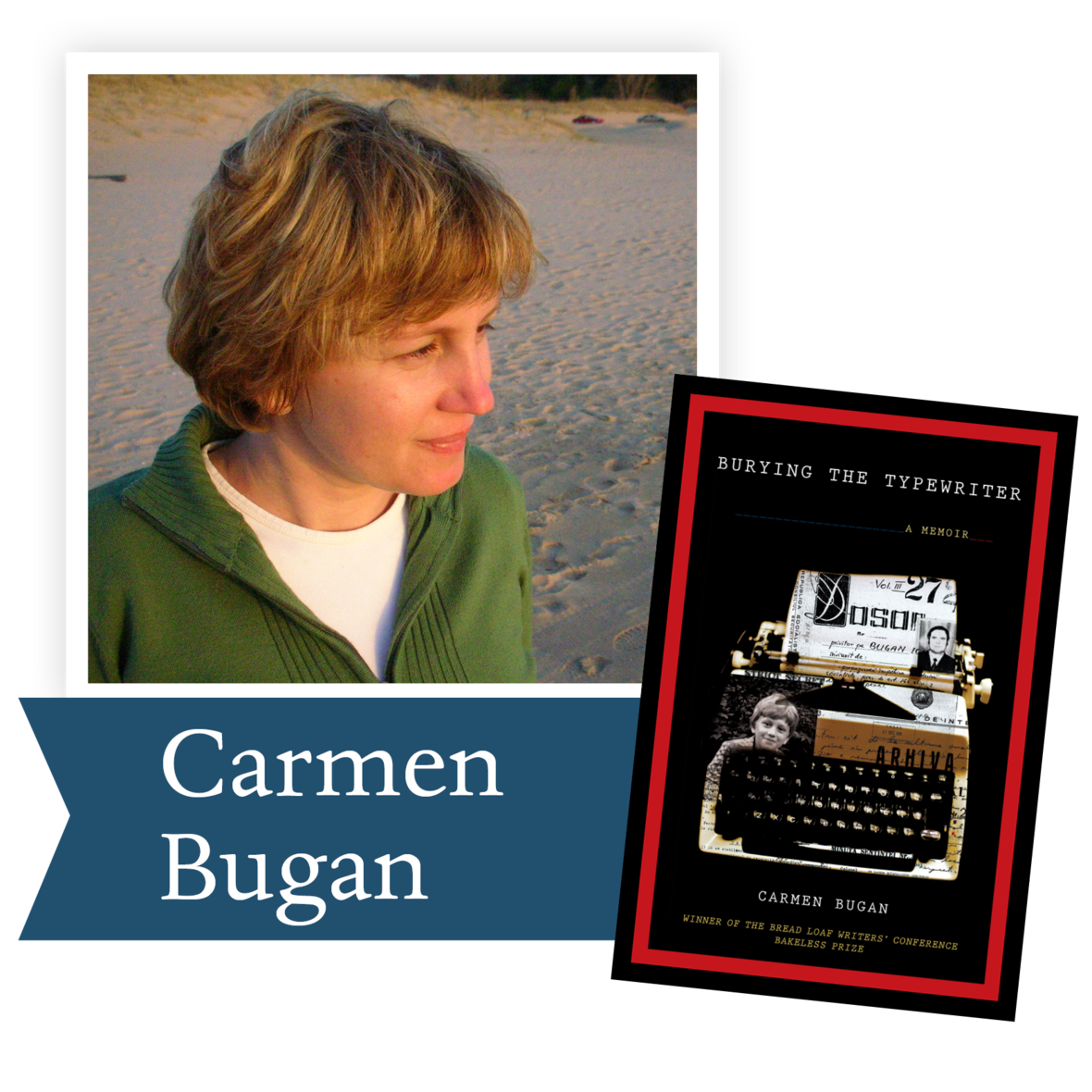Burying the Typewriter by Carmen Bugan
(Graywolf Press): In this debut memoir, a Romanian girl’s bucolic life is upended when her father is arrested for political dissidence.

 table div table+table+table+table+table+table+table+table+table+table+table+table+table+table+table+table+table+table+table+table+table+table+table+table+table+table+table+table div table{width:100%;padding:0}table div table+table+table+table+table+table+table+table+table+table+table+table+table+table+table+table+table+table+table+table+table+table+table+table+table+table+table+table div table img{width:96.23%;padding:0;float:none}table div table+table+table+table+table+table+table+table+table+table+table+table+table+table+table+table+table+table+table+table+table+table+table+table+table+table+table+table div table td{width:100%;padding:0 1.88% 18px}/* styles */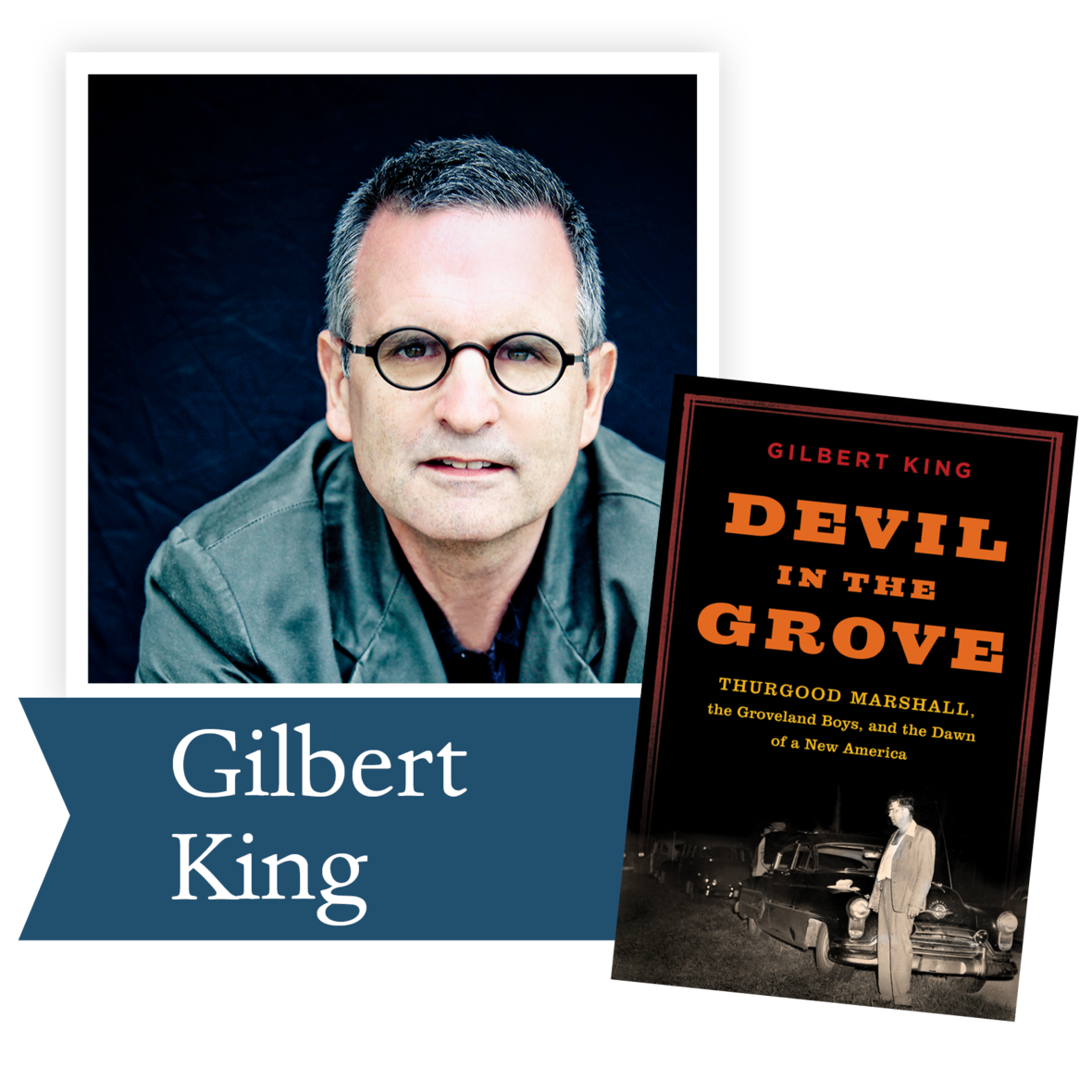Devil in the Grove by Gilbert King
(HarperCollins): A richly detailed chronicle of
four black Florida men who, falsely accused of rape in 1949, were defended by civil rights crusader Thurgood Marshall—later the first African- American Supreme Court justice.

 table div table+table+table+table+table+table+table+table+table+table+table+table+table+table+table+table+table+table+table+table+table+table+table+table+table+table+table+table+table+table div table{width:100%;padding:0}table div table+table+table+table+table+table+table+table+table+table+table+table+table+table+table+table+table+table+table+table+table+table+table+table+table+table+table+table+table+table div table img{width:96.23%;padding:0;float:none}table div table+table+table+table+table+table+table+table+table+table+table+table+table+table+table+table+table+table+table+table+table+table+table+table+table+table+table+table+table+table div table td{width:100%;padding:0 1.88% 18px}/* styles */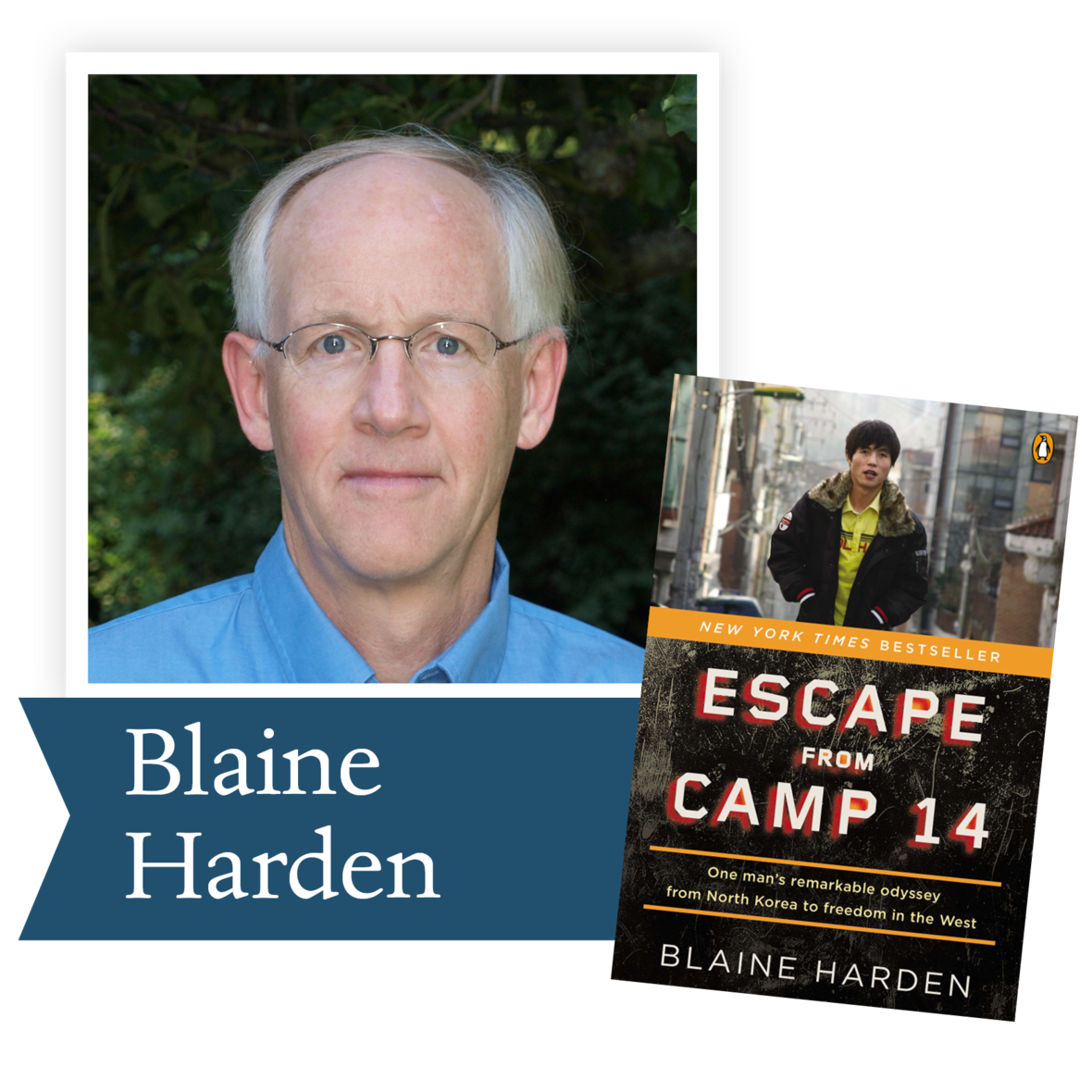Escape from Camp 14 by Blaine Harden (Viking): Bred to be a slave and a snitch, Shin Dong-hyuk is the only known person born in a North Korean prison camp to escape and survive. This bestselling account inspired a UN investigation of such camps earlier this year.

 table div table+table+table+table+table+table+table+table+table+table+table+table+table+table+table+table+table+table+table+table+table+table+table+table+table+table+table+table+table+table+table+table div table{width:100%;padding:0}table div table+table+table+table+table+table+table+table+table+table+table+table+table+table+table+table+table+table+table+table+table+table+table+table+table+table+table+table+table+table+table+table div table img{width:96.23%;padding:0;float:none}table div table+table+table+table+table+table+table+table+table+table+table+table+table+table+table+table+table+table+table+table+table+table+table+table+table+table+table+table+table+table+table+table div table td{width:100%;padding:0 1.88% 18px}/* styles */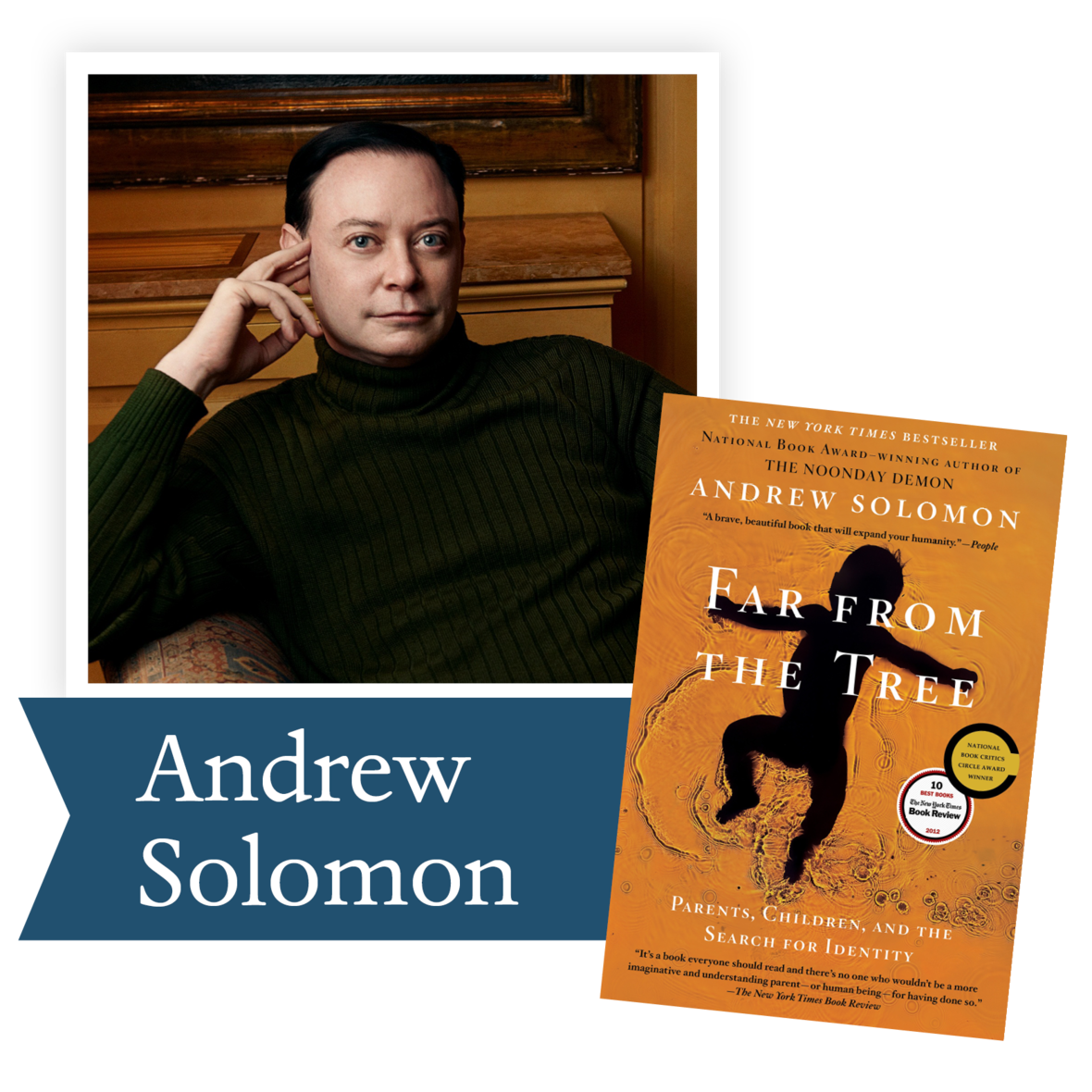Far From the Tree by Andrew Solomon (Scribner): In telling the stories of exceptional children affected by a spectrum of cognitive, physical or psychological differences, Solomon uncovers the intense prejudice they face and meets the parents who embrace their differences and try to alter the world’s understanding of their conditions.

 table div table+table+table+table+table+table+table+table+table+table+table+table+table+table+table+table+table+table+table+table+table+table+table+table+table+table+table+table+table+table+table+table+table+table div table{width:100%;padding:0}table div table+table+table+table+table+table+table+table+table+table+table+table+table+table+table+table+table+table+table+table+table+table+table+table+table+table+table+table+table+table+table+table+table+table div table img{width:96.23%;padding:0;float:none}table div table+table+table+table+table+table+table+table+table+table+table+table+table+table+table+table+table+table+table+table+table+table+table+table+table+table+table+table+table+table+table+table+table+table div table td{width:100%;padding:0 1.88% 18px}/* styles */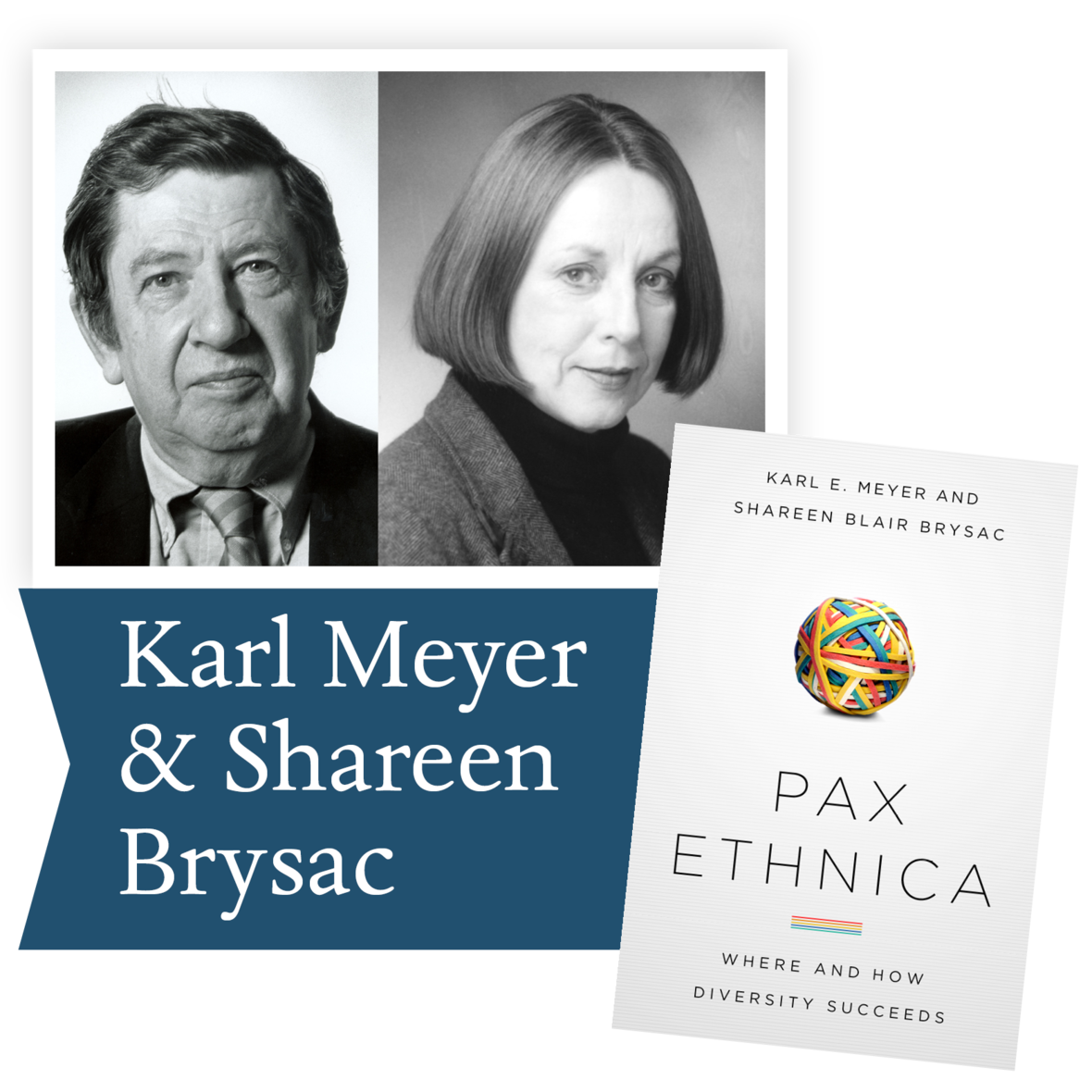Pax Ethnica by Karl Meyer and Shareen Brysac (Public Affairs Books): From Kerala, India to Queens, New York, the authors explore regions noted for low violence, rising life expectancy, and pragmatic compromises on cultural rights, revealing how diverse communities manage to live in peace.

 table div table+table+table+table+table+table+table+table+table+table+table+table+table+table+table+table+table+table+table+table+table+table+table+table+table+table+table+table+table+table+table+table+table+table+table+table div table{width:100%;padding:0}table div table+table+table+table+table+table+table+table+table+table+table+table+table+table+table+table+table+table+table+table+table+table+table+table+table+table+table+table+table+table+table+table+table+table+table+table div table img{width:96.23%;padding:0;float:none}table div table+table+table+table+table+table+table+table+table+table+table+table+table+table+table+table+table+table+table+table+table+table+table+table+table+table+table+table+table+table+table+table+table+table+table+table div table td{width:100%;padding:0 1.88% 18px}/* styles */## 2013 Ambassador Richard C. Holbrooke Distinguished Achievement Award WinnerIn the thousands of Google alerts about our authors, no one is quoted or referenced more than Wendell Berry. The variety of sources that quote him shows the expanse of his talent as he is revered by environmentalists, ministers, sociologists, farmers, poets, novelists, essayists, political activists and his neighbors. His work embodies the vast expanses of human experience with a simplicity that is elegant and profound. If you have not picked up a book by Wendell Berry, this is a perfect time to get to know him.

This newsletter’s suggestion comes from a writer for National Catholic Register, Jason Craig, who recommends novels by Wendell Berry.

By becoming unbound in the name of freedom we’ve become divorced from all sorts of things — most notably, each other.
Jason Craig

So here are some ideas for reading in the genre of “things that are really making us sick,” with a special eye toward social isolation, a thing we were experiencing long before any virus:

I don’t read enough novels, but Jayber Crow by Wendell Berry is one that I’ll pick up over and over. Berry is known for various reasons – poetry, essays, novels – but if he is known, it is known he writes from a quintessentially agrarian point of view. He watched firsthand his own farm-based community unravel in the wake of economic and technological changes, and he has painfully shown us in books like The Unsettling of America what we really lost when we lost our local cultures.

Some dismiss such views with a sort of flippancy (“What, you don’t like cheap tomatoes available whenever you want some?”), but anyone familiar with Berry’s work knows his penetrating and realistic observations are anything but romanticism. With the loss of local cultures, we lost more than our agrarian backbones and strong hands, because by becoming unbound in the name of freedom we’ve become divorced from all sorts of things — most notably, each other.

Jayber Crow follows Jayber (not his real name), an essentially orphaned boy, as he moves from belonging to disorientation, from the pseudo-freedom a young man thinks he wants to re-finding home, place and people. As I’ve written elsewhere, we can think of Jayber as a sort of priest, since he eventually makes an offering of himself for love that even results in a voluntary celibacy. He cleans the local church and even digs the graves for the dead. His main work of barbering, as one of his customers puts it, is not really a “line of work” but more of a position held, a vocation. In a time when belonging and place are things we simply don’t possess, and work is a means of paying bills but void of meaning, Jayber Crow is a potent read. In a time of social distancing, this novel will remind us how important the people near us really are. (If you wanted a Berry novel that is a bit shorter, however, you can grab Hannah Coulter, which writes of the same community but from a different lens, and it’s a bit shorter.)

 table div table+table+table+table+table+table+table+table+table+table+table+table+table+table+table+table+table+table+table+table+table+table+table+table+table+table+table+table+table+table+table+table+table+table+table+table+table+table+table div table{width:100%;padding:0}table div table+table+table+table+table+table+table+table+table+table+table+table+table+table+table+table+table+table+table+table+table+table+table+table+table+table+table+table+table+table+table+table+table+table+table+table+table+table+table div table img{width:96.23%;padding:0;float:none}table div table+table+table+table+table+table+table+table+table+table+table+table+table+table+table+table+table+table+table+table+table+table+table+table+table+table+table+table+table+table+table+table+table+table+table+table+table+table+table div table td{width:100%;padding:0 1.88% 18px}/* styles */table div table+table+table+table+table+table+table+table+table+table+table+table+table+table+table+table+table+table+table+table+table+table+table+table+table+table+table+table+table+table+table+table+table+table+table+table+table+table+table+table+table div table{width:100%;padding:0}table div table+table+table+table+table+table+table+table+table+table+table+table+table+table+table+table+table+table+table+table+table+table+table+table+table+table+table+table+table+table+table+table+table+table+table+table+table+table+table+table+table div table img{width:96.23%;padding:0;float:none}table div table+table+table+table+table+table+table+table+table+table+table+table+table+table+table+table+table+table+table+table+table+table+table+table+table+table+table+table+table+table+table+table+table+table+table+table+table+table+table+table+table div table td{width:100%;padding:0 1.88% 18px}/* styles */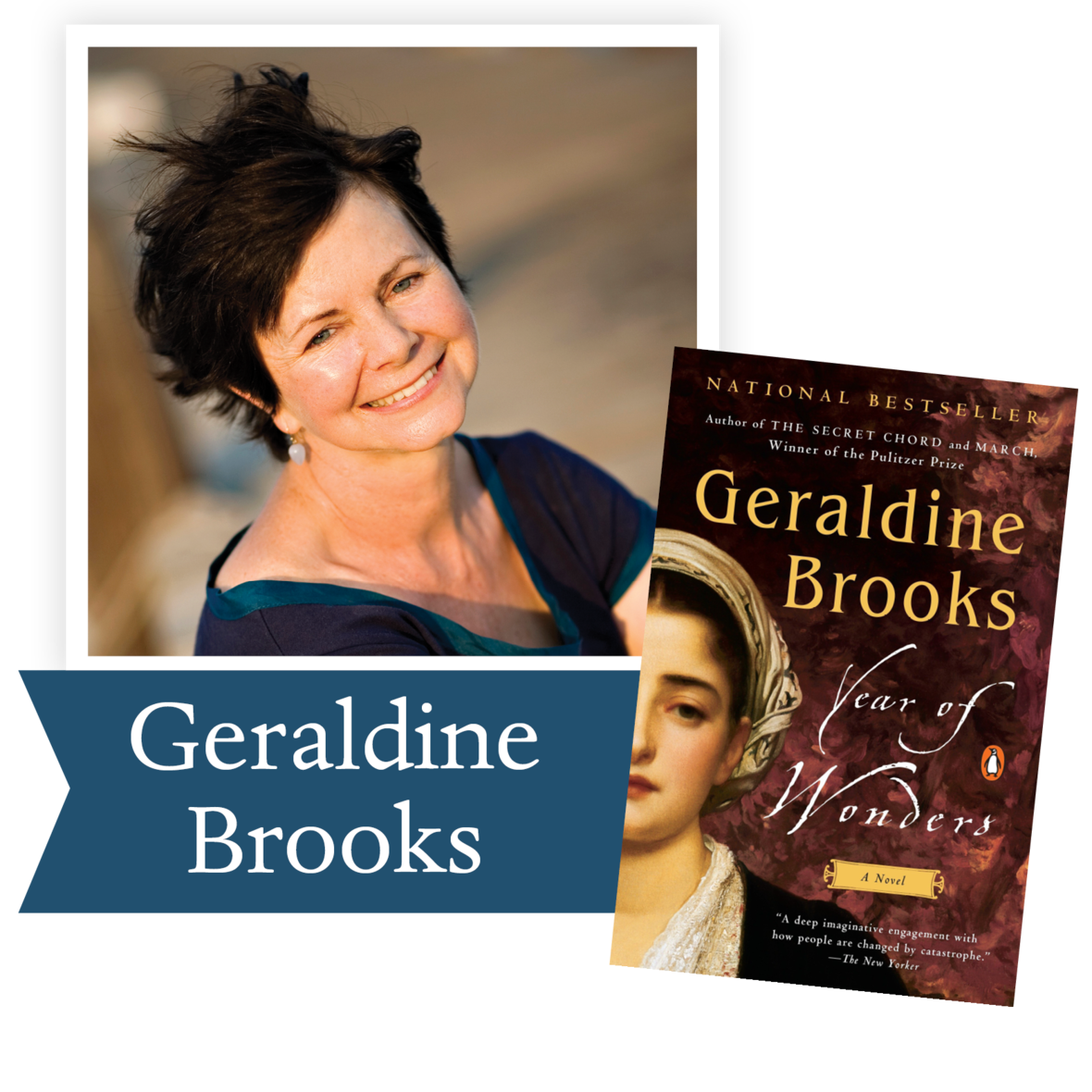“Plague stories remind us that we cannot manage without community . . . Year of Wonders is a testament to that very notion.” – The Washington Post

An unforgettable tale, set in 17th century England, of a village that quarantines itself to arrest the spread of the plague, from the author of The Secret Chord and of March, winner of the Pulitzer Prize.

Ron Rollins, Dayton Daily News Ideas and Voices Editor, will moderate the discussion of The Year of Wonders.

Join us TONIGHT, Wednesday, May 20 at 7:30 pm via Zoom for our first book club! Email Emily Kretzer to be added to the list.

 table div table+table+table+table+table+table+table+table+table+table+table+table+table+table+table+table+table+table+table+table+table+table+table+table+table+table+table+table+table+table+table+table+table+table+table+table+table+table+table+table+table+table+table div table{width:100%;padding:0}table div table+table+table+table+table+table+table+table+table+table+table+table+table+table+table+table+table+table+table+table+table+table+table+table+table+table+table+table+table+table+table+table+table+table+table+table+table+table+table+table+table+table+table div table img{width:96.23%;padding:0;float:none}table div table+table+table+table+table+table+table+table+table+table+table+table+table+table+table+table+table+table+table+table+table+table+table+table+table+table+table+table+table+table+table+table+table+table+table+table+table+table+table+table+table+table+table div table td{width:100%;padding:0 1.88% 18px}/* styles */Support the Dayton Literary Peace Prize when you shop with Amazon using this link.